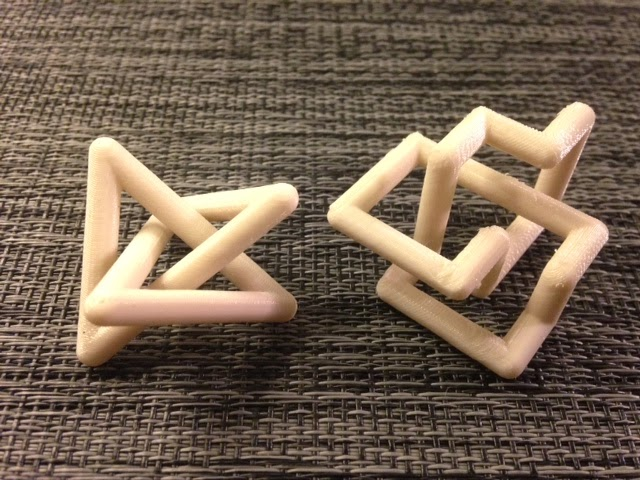# Day 153 – Stick and lattice trefoil knots## Day 153 – Stick and lattice trefoil knots

Day 153 – Stick and lattice trefoil knots 640 480 mathgrrl

To finish our collection of trefoil conformations, we have a stick knot and a cubic lattice knot:

STL file for stick: http://www.geekhaus.com/makerhome/day153_trefoil_stick_40_25.stl
STL file for lattice: http://www.geekhaus.com/makerhome/day153_trefoil_lattice_40_25.stl

Technical notes, math flavor: Usually in knot theory, although the knots we draw are curvy, in the back of our minds we require that the knots we study are nice enough to be put into some piecewise-linear format with a finite number of straight pieces. This is to avoid having to deal with wild knots, which are truly unreasonable and the sort of thing you see in the following diagram from Rolfsen’s book Knots and Links (image here from Aaron Heap of SUNY Geneseo):

I remember when I first saw this picture, at literally half the age I am now, while in an REU program learning about knot theory for the first time. My brain fell into a thousand tiny pieces, after which I agreed: we *definitely* do not want to work with knots like these. (Nowadays we have fancier pictures of the terrible and wonderful Alexander horned sphere, if you are interested.)

Anyway, the stick and lattice knots above are very nice knots, not wild at all, but the first question we have to ask about such knots is this: given a knot, can we always represent it in such a fashion? And if so, what is the fewest number of sticks that we can use (the stick number)? In the first example we have a stick knot for which the only requirement is that the sticks be sticks. In the second picture we also require that the sticks sit on a cubic integer lattice, which means that more sticks are required. People also study knots made out of sticks that are all the same length (“equilateral” stick knots) and knots that are made out of sticks with the same length and the same angle between each adjacent pair of sticks (“alpha-regular” stick knots). The stick knot model of the trefoil above illustrates that 6 is the minimum possible number of sticks that can be used to make a trefoil. The right-angled lattice knot on the right side of the picture illustrates that 24 units is the minimum length of rope that can be used to make the trefoil in the cubic lattice.

Technical notes, OpenSCAD flavor: The code for these knots is easy; each knot is constructed from a very small number of spheres at specific coordinates which are combined in “hulls” when adjacent. The coordinate data is from The Knot Server. And yes, this is ridiculously stupid OpenSCAD code but it makes pretty knots anyway!

// trefoil in minimal stick conformation
// http://newweb.cecm.sfu.ca/cgi-bin/KnotPlot/KnotServer/kserver?ncomp=1&ncross=3&id=1
s = 13;
r=2.5;
p1=[s*0.300775, s*1.301248, s*(-0.702434)];
p2=[s*(-0.976281), s*(-0.910795), s*0.701983];
p3=[s*0.976171, s*(-0.910795), s*(-0.702076)];
p4=[s*(-0.300495), s*1.300967, s*0.702620];
p5=[s*(-1.276451), s*(-0.390204), s*(-0.702474)];
p6=[s*1.276282, s*(-0.390420), s*0.702381];
hull(){
translate(p1) sphere(r);
translate(p2) sphere(r);
}
hull(){
translate(p2) sphere(r);
translate(p3) sphere(r);
}
hull(){
translate(p3) sphere(r);
translate(p4) sphere(r);
}
hull(){
translate(p4) sphere(r);
translate(p5) sphere(r);
}
hull(){
translate(p5) sphere(r);
translate(p6) sphere(r);
}
hull(){
translate(p6) sphere(r);
translate(p1) sphere(r);
}

// trefoil as minimal cubic lattice knot
// http://www.knotplot.com/EquiLat/CubicLatticeKnots.html
// http://bit-player.org/wp-content/extras/bph-publications/AmSci-1997-11-Hayes-square-knots/compsci9711.html
s = 11;
r=2.5;
p1=[s*0, s*0, s*2];
p2=[s*0, s*2, s*2];
p3=[s*2, s*2, s*2];
p4=[s*2, s*1, s*2];
p5=[s*3, s*1, s*2];
p6=[s*3, s*1, s*0];
p7=[s*1, s*1, s*0];
p8=[s*1, s*1, s*3];
p9=[s*2, s*1, s*3];
p10=[s*2, s*2, s*3];
p11=[s*3, s*2, s*3];
p12=[s*3, s*2, s*1];
p13=[s*2, s*2, s*1];
p14=[s*2, s*0, s*1];
p15=[s*1, s*0, s*1];
p16=[s*1, s*0, s*2];
p17=[s*0, s*0, s*2];
translate([-1.5*s,-1.5*s,-1.5*s])
union(){
hull(){
translate(p1) sphere(r);
translate(p2) sphere(r);
}
hull(){
translate(p2) sphere(r);
translate(p3) sphere(r);
}
hull(){
translate(p3) sphere(r);
translate(p4) sphere(r);
}
hull(){
translate(p4) sphere(r);
translate(p5) sphere(r);
}
hull(){
translate(p5) sphere(r);
translate(p6) sphere(r);
}
hull(){
translate(p6) sphere(r);
translate(p7) sphere(r);
}
hull(){
translate(p7) sphere(r);
translate(p8) sphere(r);
}
hull(){
translate(p8) sphere(r);
translate(p9) sphere(r);
}
hull(){
translate(p9) sphere(r);
translate(p10) sphere(r);
}
hull(){
translate(p10) sphere(r);
translate(p11) sphere(r);
}
hull(){
translate(p11) sphere(r);
translate(p12) sphere(r);
}
hull(){
translate(p12) sphere(r);
translate(p13) sphere(r);
}
hull(){
translate(p13) sphere(r);
translate(p14) sphere(r);
}
hull(){
translate(p14) sphere(r);
translate(p15) sphere(r);
}
hull(){
translate(p15) sphere(r);
translate(p16) sphere(r);
}
hull(){
translate(p16) sphere(r);
translate(p17) sphere(r);
}
hull(){
translate(p17) sphere(r);
translate(p1) sphere(r);
}
}

——————

As an Amazon Associate we earn from qualifying purchases, so if you’ve got something you need to pick up anyway, going to Amazon through this linkwill help us keep Hacktastic running. Thanks! :)